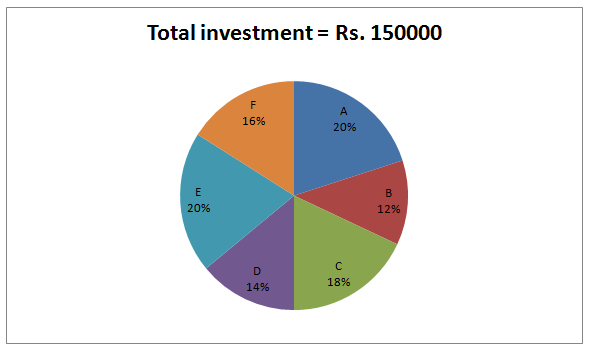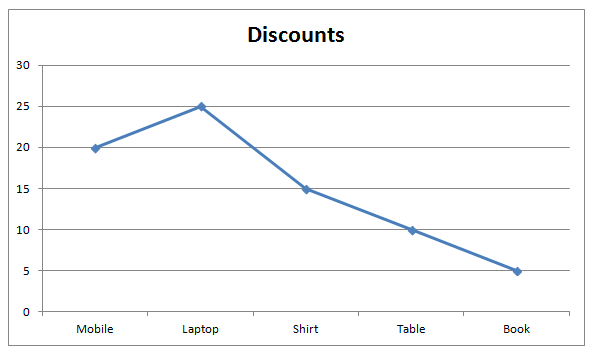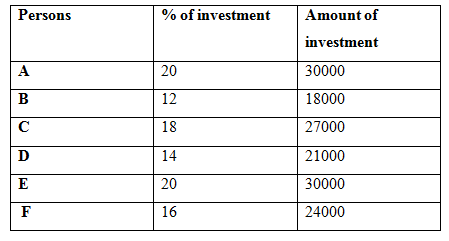# SBI PO Quantitative Aptitude Questions 2019 (Day-9) High Level New Pattern

SBI PO 2019 Notification is about to come and it is the most awaited exam among the aspirants. We all know that new pattern questions are introducing every year in the SBI PO exam. Further, the questions are getting tougher and beyond the level of the candidate’s expectations.

Our IBPS Guide is providing High-Level New Pattern Quantitative Aptitude Questions for SBI PO 2019 so the aspirants can practice it on a daily basis. These questions are framed by our skilled experts after understanding your needs thoroughly. Aspirants can practice these high-level questions daily to familiarize with the exact exam pattern. We wish that your rigorous preparation leads you to a successful target of becoming SBI PO.

[WpProQuiz 4808]

### Click Here for SBI PO Pre 2019 High-Quality Mocks Exactly on SBI Standard

Directions (1 – 5): Study the following information carefully and answer the given questions.

The following pie chart shows the percentage distribution of total investment of six different persons.1) A and C started a business. After 5 months, A invested Rs. 4000 more and at the same time G enter into a business by investing Rs. 5000 more than the investment of C. Find the share of G, if the total share of A and C together is Rs. 103240?

a) Rs. 35620

b) Rs. 48950

c) Rs. 32480

d) Rs. 41230

e) None of these

2) B and D together started a business. After 6 months, B left the business and at the same time E enters into a business. D left the business after 2 months of B left the business. E invested the amount for x months only. Out of the total profit of Rs. 72000 for the certain period, D received the share of Rs. 21000. Find the value of x?

a) 10

b) 9

c) 12

d) 8

e) None of these

3) B, F and N together started a business by investing in the ratio of 3: 4 : 6. After 7 months, N is replaced by D. And after another 2 months, B invested Rs. 6000 more. Find the total profit at the end of the year, if the share of B and D together is Rs. 113000?

a) Rs. 278000

b) Rs. 252000

c) Rs. 316000

d) Rs. 293000

e) None of these

4) B, E and F started a business. B is a working partner but E and F are sleeping partner in the business. For managing the business, B received 10% of the total profit. Find the difference between the share of B and F, if the total profit at the end of the year is Rs. 78000?

a) Rs. 2350

b) Rs. 1950

c) Rs. 2500

d) Rs. 1600

e) None of these

5) C, D and E together started a business. After 4 months, C withdraw one-third of the initial investment and after another 2 months, E withdraw one-sixth of the initial investment. Find the share of E, if the total profit at the end of the year is Rs. 139000?

a) Rs. 63000

b) Rs. 48000

c) Rs. 55000

d) Rs. 52000

e) None of these

Direction (6-10): Study the following information carefully and answer the given questions:

The given line graph shows the discount percent of five different items-Mobile, Laptop, Shirt, Table and Book.6) Mani buys a washing machine with a marked price of Rs.14000 at a certain discount. He also buys a table, having a marked price of Rs.500. He gains an extra 8% discount on the total purchase price of washing machine and table. If he ended up paying Rs.12000, then what was the approximate discount on the washing machine?

a) 14%

b) 10%

c) 15%

d) 25%

e) 28%

7) If Shon went to shop and purchased mobile and laptop. Marked price of mobile is Rs. 4500 and the cost price of the laptop is Rs.10000. If shopkeeper marked both the items 20% above the cost price, then what is the overall approximate percent profit or loss of Shon in this transaction?

a) 16%

b) 8%

c) 23%

d) 13%

e) 15%

8) Marked price of the shirt is Rs.480. Shopkeeper mistakenly calculated the discount twice and still makes a profit of 20%. Find the approximate profit that would have been earned by the shopkeeper if he had calculated the discount once?

a) 38%

b) 41%

c) 47%

d) 48%

e) 33%

9) If the marked up price of Book is 50% above the cost price of the book. Total amount of discount offered on Book is Rs.150 and the profit of book is Rs.850. If the shopkeeper interchanges the marked up percent and discount percent offered on book, then what is the difference between original selling price and new selling price of Book?

a) Rs.1800

b) Rs.1960

c) Rs.1260

d) Rs.1360

e) None of these

10) A retailer bought a mobile. He sold the mobile to the customer for Rs.26000 and earned a profit of 30% of his cost price. What was the initial marked price of the mobile?

a) Rs.25000

b) Rs.18000

c) Rs.21000

d) Rs.24000

e) None of these

Directions (1 -5):The investment of G = 27000 + 5000 = Rs. 32000

The share of A, C and G,

= > [30000*5 + 34000*7]: [27000*12]: [32000*7]

= > [150000 + 238000]: 324000: 224000

= > 388000: 324000: 224000

= > 97: 81: 56

The total share of A and C together = Rs. 103240

178’s = 103240

1’s = 580

The share of G = 56’s = Rs. 32480

The share of D = Rs. 21000

The share of B and E = 72000 – 21000 = Rs. 51000

The share of B, D and E,

= > [1500*12*6] : [1500*14*8] : [1500*20*x]

= > 18 : 28 : 5x

According to the question,

= > 28/(18 + 5x) = 21000/51000

= > 5x = 50

= > x = 10

B, F and N together started a business by investing in the ratio of = 3: 4: 6

The investment of N = (24000/4)*6 = Rs. 36000

The share of B, F, N and D is,

= > [18000*9 + 24000*3] : [24000*12] : [36000*7] : [21000*5]

= > 234000: 288000: 252000: 105000

= > 78: 96: 84: 35

The share of B and D together = Rs. 113000

113’s = 113000

1’s = 1000

Total profit = 293’s = Rs. 293000

The share of B, E and F

= > 18000: 30000: 24000

= > 3: 5: 4

B = 78000*(10/100) = Rs. 7800

The share of B = 7800 + (70200/12)*3 = 7800 + 17550 = Rs. 25350

The share of E = (70200/12)*5 = Rs. 29250

The share of F = (70200/12)*4 = Rs. 23400

Required difference = 25350 – 23400 = Rs. 1950

The share of C, D and E

= > [27000*4 + 27000*(2/3)*8]: [21000*12]: [30000*6 + 30000*(5/6)*6]

= > [108000 + 144000]: : [180000 + 150000]

= > 252000: 252000: 330000

= > 84: 84: 110 = 42: 42: 55

139’s = 139000

1’s = 1000

The share of E = 55’s = Rs. 55000

Table=500*90/100=450

Amount paid=92/100*(((100-x)/100*14000)+450)

12000=(92/100)(140(100-x)+450)

12000=(92/100)(14000-140x+450)

12000=92/100(14450-140x)

1200000=1329400-12880x

12880x = 129400

X=10%

Marked price of mobile=Rs.4500

Selling price of mobile=4500*80/100=Rs.3600

Cost price of mobile=4500*100/120=3750

Cost price of laptop=Rs.10000

Marked price of laptop = Rs.10000 * 120/100 = 12000

Selling price of laptop=12000*75/100=9000

Total cost price=3750+10000=13750

Total selling price=3600+9000=12600

Required loss percent=(13750-12600)/13750*100≈8%

Discount twice=480*85/100*85/100=Rs.346.8

CP he makes 20% profit=346.8*100/120=289

SP of discount allows once=480*85/100=408

Profit percentage= (408-289)/289*100=41%

Cost price = 100x

Marked price of Book=150x

Selling price of Book=95/100*150x=142.5x

Discount amount=150x-142.5x=150

7.5x=150

X=20

Cost price of Book=100x =2000

MP of Book=150*20=3000

SP of Book=142.5*20=2850

After interchange of marked up percentage and discount percentage,

MP of Book=105/100*2000=2100

SP of Book=(100-50)/100*2100=1050

Difference=2850-1050= Rs.1800

CP of mobile=26000*100/130=20000

Marked price=20000*100/80=25000

### Click Here for SBI PO Pre 2019 High-Quality Mocks Exactly on SBI Standard

Daily Practice Test Schedule | Good Luck

 Topic Daily Publishing Time Daily News Papers & Editorials 8.00 AM Current Affairs Quiz 9.00 AM Current Affairs Quiz (Hindi) 9.30 AM NIACL AO Prelims – Reasoning 10.00 AM NIACL AO Prelims – Reasoning (Hindi) 10.30 AM NIACL AO Prelims – Quantitative Aptitude 11.00 AM NIACL AO Prelims – Quantitative Aptitude (Hindi) 11.30 AM Vocabulary (Based on The Hindu) 12.00 PM NIACL AO Prelims – English Language 1.00 PM SSC Practice Questions (Reasoning/Quantitative aptitude) 2.00 PM IBPS Clerk – GK Questions 3.00 PM SSC Practice Questions (English/General Knowledge) 4.00 PM Daily Current Affairs Updates 5.00 PM SBI PO/IBPS Clerk Mains – Reasoning 6.00 PM SBI PO/IBPS Clerk Mains – Quantitative Aptitude 7.00 PM SBI PO/IBPS Clerk Mains – English Language 8.00 PM